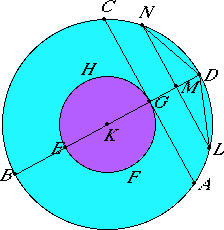# Proposition 16

Given two circles about the same center, to inscribe in the greater circle an equilateral polygon with an even number of sides which does not touch the lesser circle.

Let ABCD and EFGH be the two given circles about the same center K.

It is required to inscribe in the greater circle ABCD an equilateral polygon with an even number of sides which does not touch the circle EFGH.I.11

Draw the straight line BKD through the center K, and draw GA from the point G at right angles to the straight line BD, and carry it through to C.

III.16,Cor

Therefore AC touches the circle EFGH.

X.1

Then, bisecting the circumference BAD, bisecting the half of it, and doing this repeatedly, we shall leave a circumference less than AD.

Let such be left, and let it be LD.

I.12

Draw LM from L perpendicular to BD, and carry it through to N. Join LD and DN.

Therefore LD equals DN.

Now, since LN is parallel to AC, and AC touches the circle EFGH, therefore LN does not touch the circle EFGH. Therefore LD and DN are far from touching the circle EFGH.

If, then, we fit into the circle ABCD straight lines equal to the straight line LD and placed repeatedly, then there is inscribed in the circle ABCD an equilateral polygon with an even number of sides which does not touch the lesser circle EFGH.

Q.E.F.

## Guide

The purpose of this construction is to separate the two concentric circles by a polygon so that a three-dimensional construction can be made in the next proposition to separate two concentric spheres.

This construction will actually generate a polygon whose number of sides is a power of 2 such as 8, 16, 32, etc. The next proposition requires a polygon where the number of sides is not just even, but a multiple of 4, which conveniently this construction generates.

Furthermore, the next proposition requires not just that the polygon not touch the inner circle, but the chords joining alternate vertices also not touch the inner circle, which again this construction satisfies.